In a previous class, we saw that the positive reals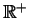is a vector space over a field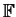(whereis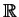or a subfield ofsuch as the rationals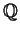), provided that we use multiplication infor vector addition, and raise our vector to the powerfor scalar multiplication by. That is, for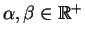and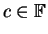, we set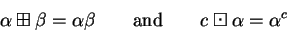In this note, we want to demonstrate that this vector space is the same as the vector space ofover(with ordinary addition and scalar multiplication). We'll continue to use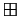to represent vector addition in, and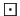to represent scalar multiplication.

First, notice that if our fieldis the real numbers, our vector spaceis a one-dimensional vector space, and so must be isomorphic towith ordinary addition (since they are both finite dimensional vector spaces over the same field and have the same dimension.)

To see that, let's find a basis for. Any positive real number other than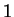will do, so let's use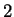. To show that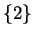is a basis, we must prove that every other element ofis a linear combination of. That means that given any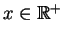, we can find a scalarfor which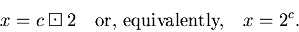But certainly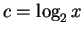is the desired solution. Since we found one vector which spans,is a one-dimensional vector space over the field.

If we use a subfield offor, then depending on which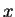we choose,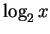may or may not be an element of, and so we will need more than one element in our basis for this other vector space. For example, if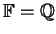, we will need an infinite basis.

The above discussion does more than show us the dimension of our vector space. It also gives us an isomorphism betweenand. To see this, we must verify that the map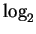is one-to-one, onto, and linear.

• If, then, and so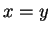.
• For any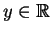, we must find anso that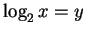. Butworks for us here, sois onto.
• At first, you might worry that the logarithm isn't a linear map. But keep in mind that it is linear with our unusual definition for addition and scalar multiplication. We have to show that for anyand for any, we have(where on the right side we are using ordinary addition and multiplication).

To see that, we just expand: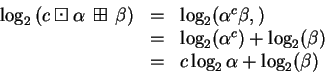as desired.

Since we have an isomorphism betweenand, we can think of these two vector spaces as the same''.

Scott Sutherland March 3, 2005